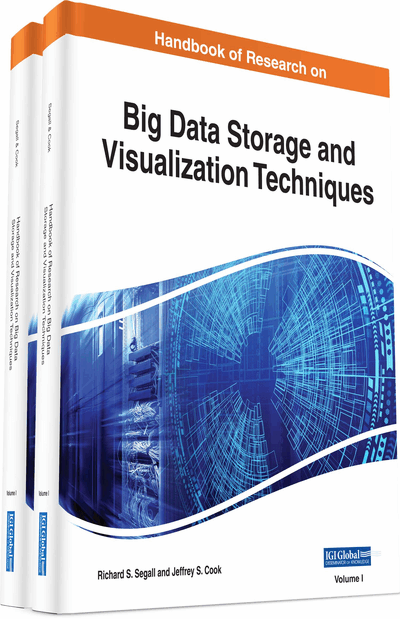# Mining Multimodal Big Data: Tensor Methods and Applications

Sujoy Roy (University of Memphis, USA) and Michael W. Berry (University of Tennessee, USA)
DOI: 10.4018/978-1-5225-3142-5.ch023
Available
\$37.50
No Current Special Offers

## Abstract

The last decade has witnessed exponential growth of data particularly in the fields of biomedicine, unstructured text processing and signal processing. There exist instances of data depicting simultaneous interactions amongst more than two types of entities. Such data are not readily amenable to matrix representation as matrices can show interactions between only two types of entities at a time. Tensors are multimodal extensions of matrices (a matrix can be thought of as 2-mode tensor), and tensor factorizations (decompositions) are multiway generalizations of matrix factorizations. This chapter provides an overview of tensor factorization methods as well as a literature review of selected applications in areas that are currently experiencing exponential data growth and likely of interest to a broad audience.
Chapter Preview
Top

## Introduction

Most datasets can be organized into tables (matrices) typically depicting pairwise relationships between two types of entities: objects (rows) and attributes (columns). The entries of the matrix contain attribute values for each object. Given a data matrixwithrows andcolumns (), theobjects can be considered-dimensional row vectors in attribute space, while theattributes may be interpreted as-dimensional column vectors in object space. The objects may be prioritized against each other or clustered together by calculating similarities between their vectors. For a large data matrix such as one containing frequencies of several thousand terms (attributes) in a few hundred documents (objects), it is time consuming to compare document vectors. Matrix factorization (decomposition) methods (Golub & Van Loan, 2012) are dimensionality reduction techniques that may be utilized in part to reduce the size of the coordinate space in which to compare the vectors.

Singular Value Decomposition (Skillicorn, 2007) is a factorization method that expresses the originalmatrix as a scaled product of,, andcomponent matrices, wheredenotes the transpose. The two sets of original object and attribute vectors are transformed into a new-dimensional orthogonal space () in which the maximum variation is expressed along the first dimension axis, as much variation independent of that is expressed along an axis orthogonal to the first, and so on. The new set of axes may reveal the true dimensionality of the data if the dataset is not inherently-dimensional. It is far less time consuming to compare object vectors in-dimensional space than in-dimensional space. Another factorization method known as Non-negative Matrix Factorization (Lee & Seung, 1999) constrains the component matrices to non-negative values in order to aid the interpretation of axes (columns) of the component matrices, and has been utilized in bi-clustering objects and attributes.

## Key Terms in this Chapter

Vector: A data structure containing a collection of elements (numbers or variables). Each element is identified using a numeric index. It may be also referred to as a 1-mode array.

Matricization: The unfolding of a tensor into a 2-mode matrix.

Dimension: There is some ambiguity in the usage of this word as it may either be used to refer to the number of modes of an array e.g., a matrix being referred to as containing 2-dimensional data; or the number of indices in a specific mode, e.g., a vector with AU252: Mathtype 351 elements may be referred to as -dimensional vector. In this chapter, its usage refers to the latter case.

Tensor: A -mode array where AU253: Mathtype 354 and each element requires AU254: Mathtype 355 indices for identification. It is typically used to represent simultaneous interactions amongst 3 or more types of entities.

Mode: The number of types of indices required to refer an element of an array.

Matrix: A 2-mode array or a table where each element requires 2 indices to identify. The two indices refer to row indices and column indices. Most datasets can be organized into matrices typically depicting pair-wise relationships between two types of entities, objects (rows) and attributes (columns). The entries (elements) of the matrix may contain attribute values for each object.

Factorization: The process of expressing a matrix or a tensor as a product of 2 or more matrices.

## Complete Chapter List

Search this Book:
Reset